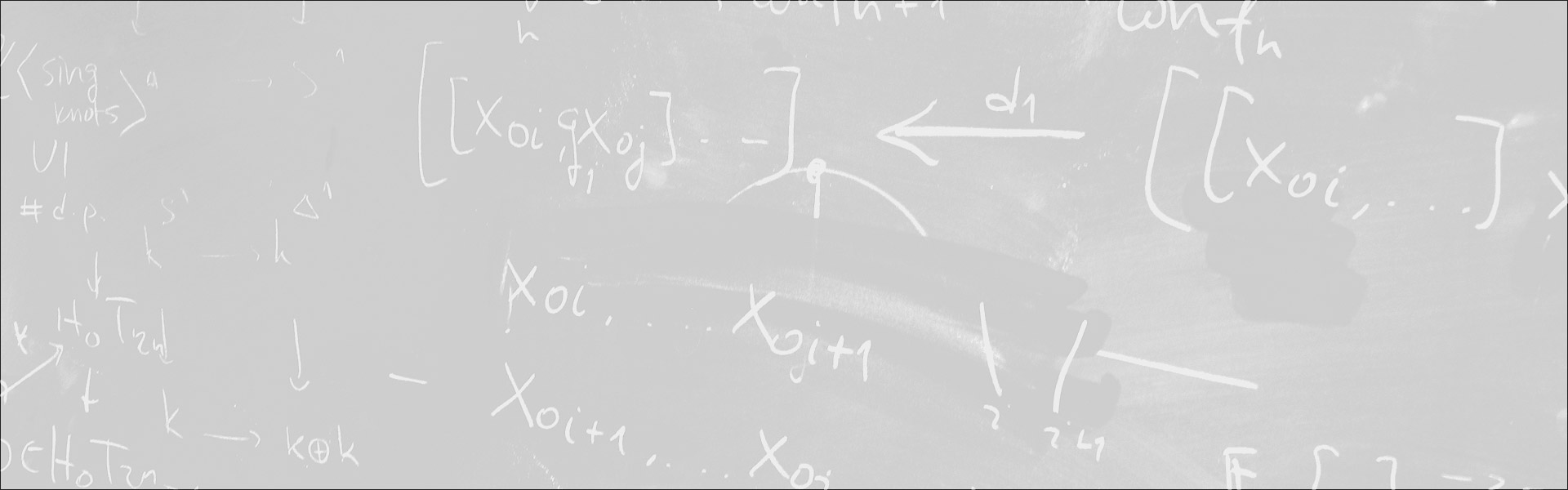Designed and built with care, filled with creative elements

Top# Satellites of spherical subgroups and Poincaré polynomials

/  Évènements• Cet évènement est passé

## Satellites of spherical subgroups and Poincaré polynomials

Let G be a connected reductive group over C. One can associate with every spherical homogeneous space G/H its lattice of weights X^*(G/H) and a subset S of M of linearly independent primitive lattice vectors which are called the spherical roots. For any subset I of S we define, up to conjugation, a spherical subgroup H_I in G such that dim H_I = dim H and X^*(G/H_I) = X^*(G/H). We call the subgroups H_I the satellites of the spherical subgroup H. Our interest in satellites H_I is motivated by the space of arcs of the spherical homogeneous space G/H.We show a close relation between the Poincaré polynomials of the two spherical homogeneous spaces G/H and G/H_I.All of this is useful for the computation of the stringy E-function of Q-Gorenstein spherical embeddings.The talk is based on joint works with Victor Batyrev.

- Séminaire Géométrie et théorie des modèles

#### Détails :

Orateur / Oratrice : Anne Moreau
Date : 10 mars 2017
Horaire : 16h00 - 16h00
Lieu : ENS Salle W